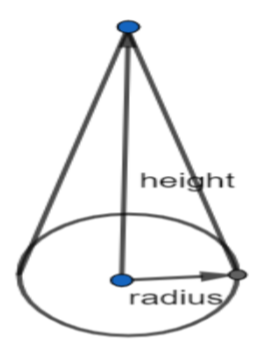QuestionAnswers

# A heap of wheat is in the form of a cone whose diameter is 10.5 m and height is 3 m. Find its volume. The heap is to be covered by canvas to protect it from rain. Find the area of the canvas required.

Hint: First of all, we should find the radius of the cone. We know that the volume of the cone is equal to V if r is the radius of the cone and h is the height of the cone, then $V=\dfrac{1}{3}\pi {{r}^{2}}h$. By using this formula, we can find the volume of the cone. We know that the curved surface area of the cone is equal to A if r is the radius of the cone, h is the height of the cone and l is the slant height of the cone, then $A=\pi rl$ where $l=\sqrt{{{r}^{2}}+{{h}^{2}}}$. By using this formula, we can find the area of the canvas.

Complete step-by-step solution:
From the question, it is clear that a heap of wheat is in the form of a cone whose diameter is 10.5 m and the height is 3 m.
We know that the volume of the cone is equal to V if r is the radius of the cone and h is the height of the cone, then $V=\dfrac{1}{3}\pi {{r}^{2}}h$.We were given that the diameter of the cone is equal to 10.5 cm.
Let us assume the diameter of the cone is equal to d.
$\Rightarrow d=10.5....(1)$
We know that if r is the radius of the cone and d is the diameter of the cone, then $d=2r$.
Now let us assume the radius of the cone is equal to r.
$\Rightarrow 10.5=2r$
By using cross multiplication, we get
\begin{align} & \Rightarrow r=\dfrac{10.5}{2} \\ & \Rightarrow r=5.25.....(2) \\ \end{align}
We were given that the height of the cone is equal to 3m.
Let us assume the height of the cone is equal to h.
$\Rightarrow h=3......(3)$
Let us assume the volume of the cone is equal to V.
We know that the volume of the cone is equal to V if r is the radius of the cone and h is the height of the cone, then $V=\dfrac{1}{3}\pi {{r}^{2}}h$.
\begin{align} & \Rightarrow V=\dfrac{1}{3}\pi {{\left( 5.25 \right)}^{2}}\left( 3 \right) \\ & \Rightarrow V=86.59.....(4) \\ \end{align}
From equation (4), it is clear that the volume of the cone is equal to $86.59c{{m}^{3}}$.
Now we should find the area of the canvas.
We know that the curved surface area of the cone is equal to A if r is the radius of the cone, h is the height of the cone and l is the slant height of the cone, then $A=\pi rl$ where $l=\sqrt{{{r}^{2}}+{{h}^{2}}}$.
So, let us assume l is the slant height of the cone.
\begin{align} & \Rightarrow l=\sqrt{{{\left( 3 \right)}^{2}}+{{\left( 5.25 \right)}^{2}}} \\ & \Rightarrow l=\sqrt{36.5625} \\ & \Rightarrow l=6.04669.....(5) \\ \end{align}
From equation (5), it is clear that the slant height of the cone is equal to 6.04669 m.
Let us assume the curved surface area of the cone is equal to A.
\begin{align} & \Rightarrow A=\pi \left( 5.25 \right)\left( 6.04669 \right) \\ & \Rightarrow A=99.756.......(6) \\ \end{align}
From equation (6), it is clear that the area of the canvas is equal to $99.756{{m}^{2}}$.

Note: Students may assume that the curved surface area of the cone is equal to A if r is the radius of the cone, h is the height of the cone, then $A=\pi rh$. Students may also assume that that the volume of the cone is equal to V if r is the radius of the cone, h is the height of the cone and l is the slant height of the cone, then $V=\dfrac{1}{3}\pi {{r}^{2}}l$ where $l=\sqrt{{{r}^{2}}+{{h}^{2}}}$. But we know that these are incorrect. So, these misconceptions should be avoided.